# Velocity vs Time Graphs The graph shows the

• Slides: 36
Download presentation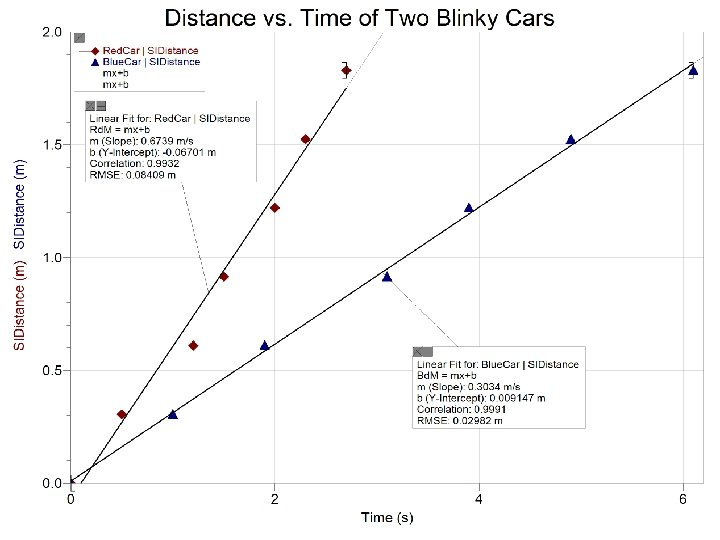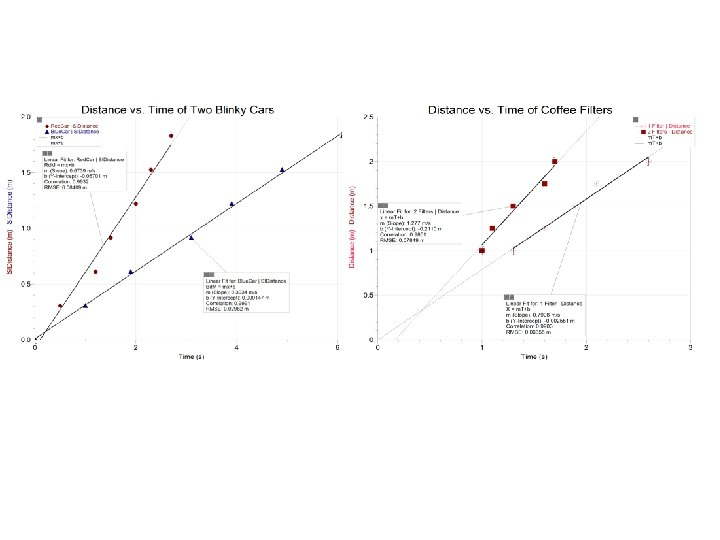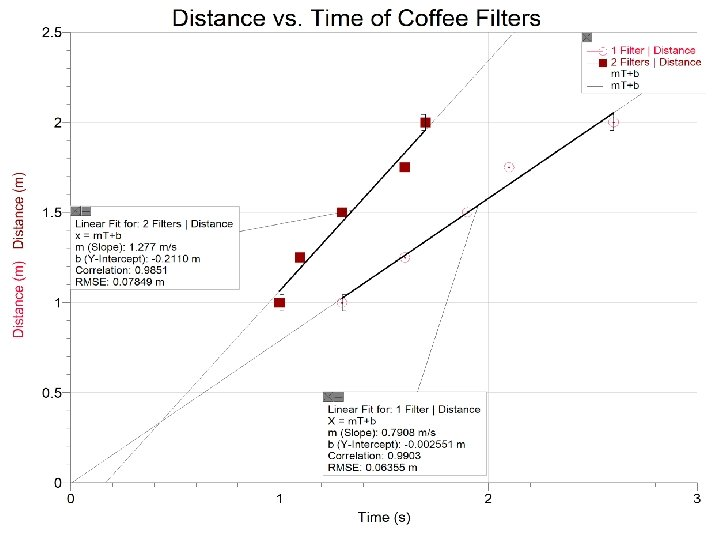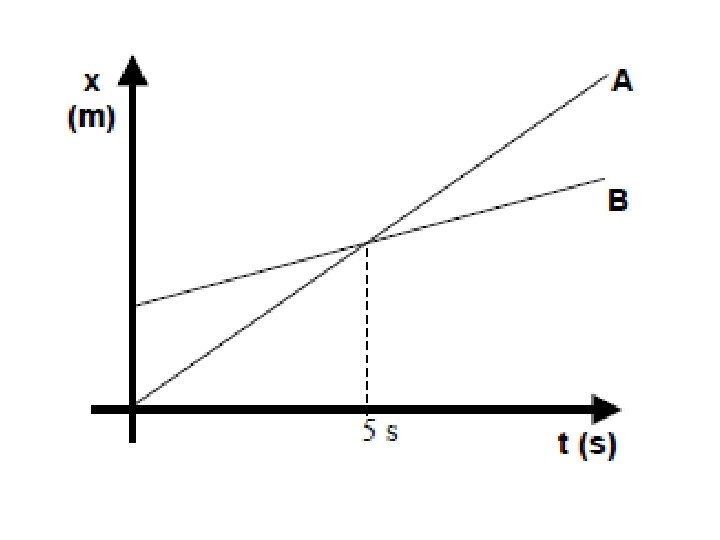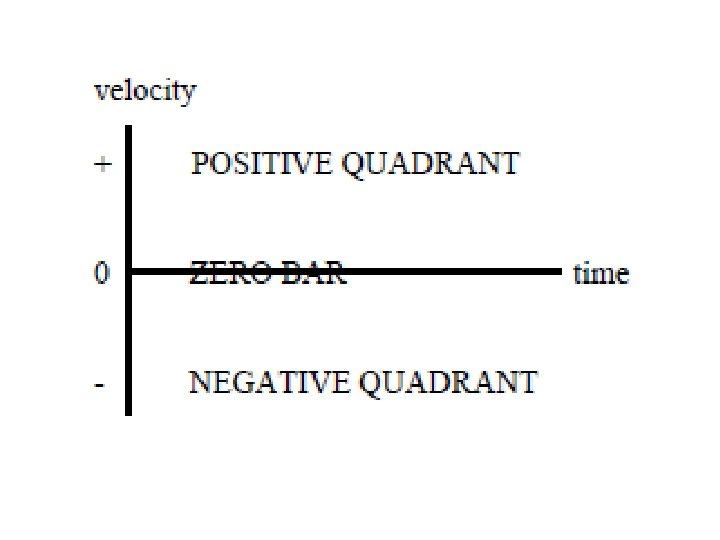Velocity vs. Time Graphs • The graph shows the velocity of an object at different times • The positive quadrant indicates that the object is moving in the forward direction • The negative quadrant indicates the object is moving in the backward direction • The zero bar indicates the object is not moving • The further the graph gets from the zero bar, the faster the object is moving • The closer the graph gets to the zero bar the slower the object is moving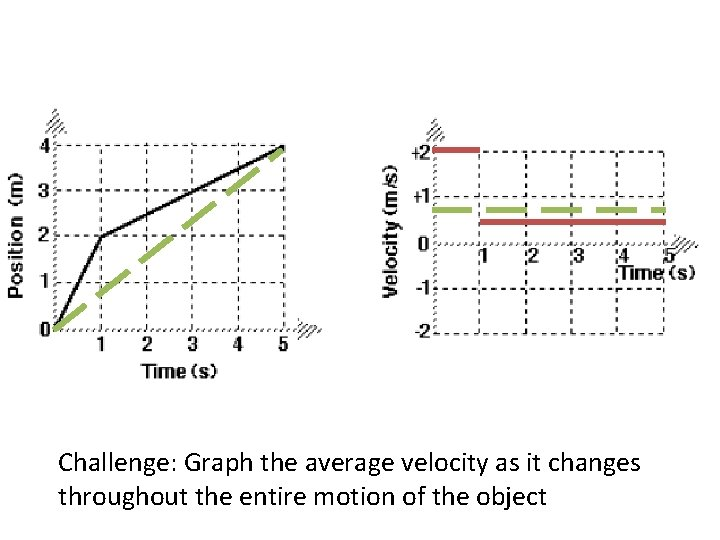Challenge: Graph the average velocity as it changes throughout the entire motion of the object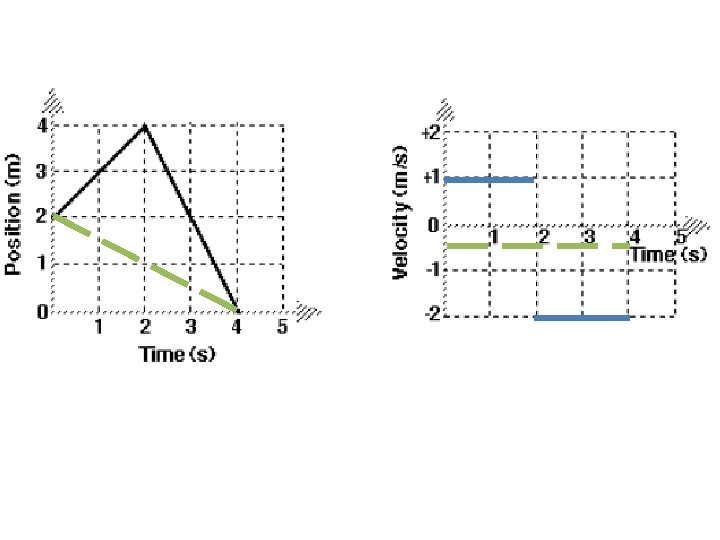Bike F Bike G • Create a position vs. time graph and a velocity vs. time graph for the bee’s motion. Include at least six “trips” from one bicycle to the other. Choose the origin to be Bike F’s position at t = 0. • Construct a distance vs. time graph and a displacement vs. time graph for the bee’s motion. • Challenge: Create a series that calculates the distance the bee travels for n trips. The nth term of the series should be the distance of the nth trip.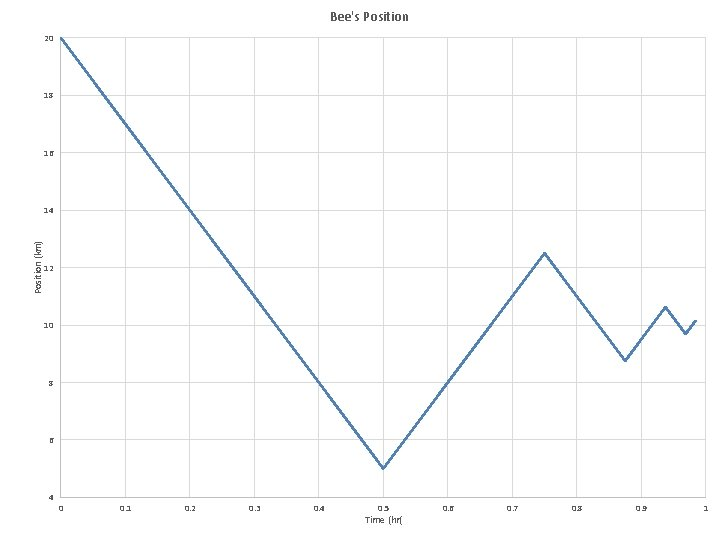Bee's Position 20 18 16 Position (km) 14 12 10 8 6 4 0 0. 1 0. 2 0. 3 0. 4 0. 5 Time (hr( 0. 6 0. 7 0. 8 0. 9 1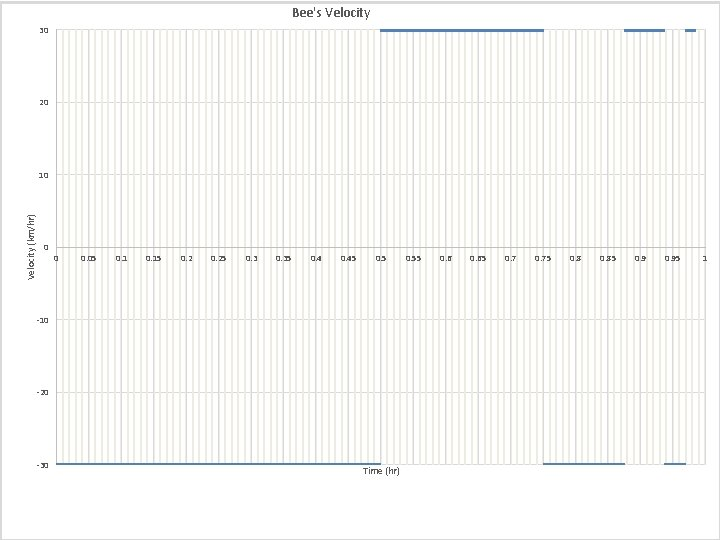Bee's Velocity 30 20 Velocity (km/hr) 10 0 0 0. 05 0. 15 0. 25 0. 35 0. 45 0. 5 -10 -20 -30 Time (hr) 0. 55 0. 65 0. 75 0. 85 0. 95 1Bee's Distance 35 30 25 Distance (km) 20 15 10 5 0 0 0. 1 0. 2 0. 3 0. 4 0. 5 Time (hr) 0. 6 0. 7 0. 8 0. 9 1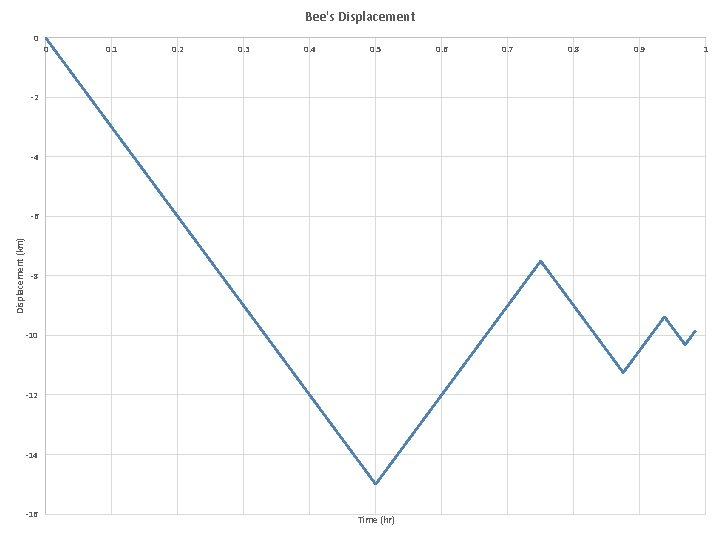Bee's Displacement 0 0 0. 1 0. 2 0. 3 0. 4 0. 5 -2 -4 Displacement (km) -6 -8 -10 -12 -14 -16 Time (hr) 0. 6 0. 7 0. 8 0. 9 1Time Progress in that second Total Displacemen t 1 2 2 4 3 2 6 4 3 9 5 3 12 6 3 15 7 3 18 8 1 19 9 1 20 10 1 21 11 -3 18 12 -3 15 13 -3 12 14 -3 9 15 -3 6 16 -3 3 17 -3 0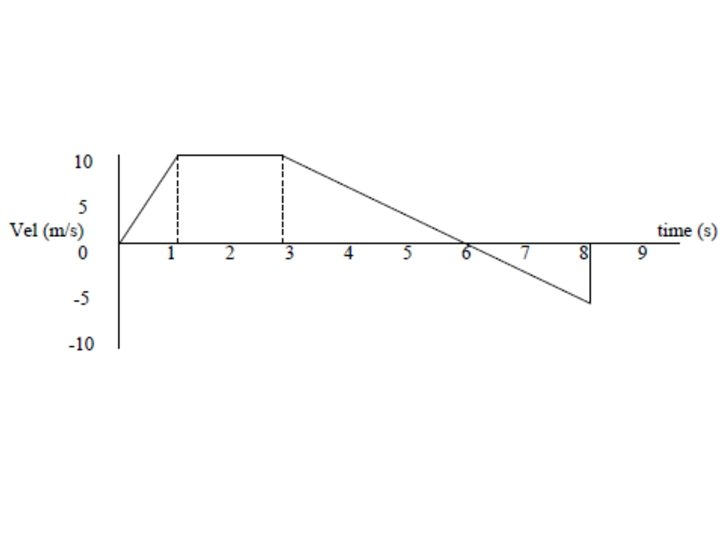• Acceleration is the rate of change of the velocity • Acceleration is the slope of a velocity vs. time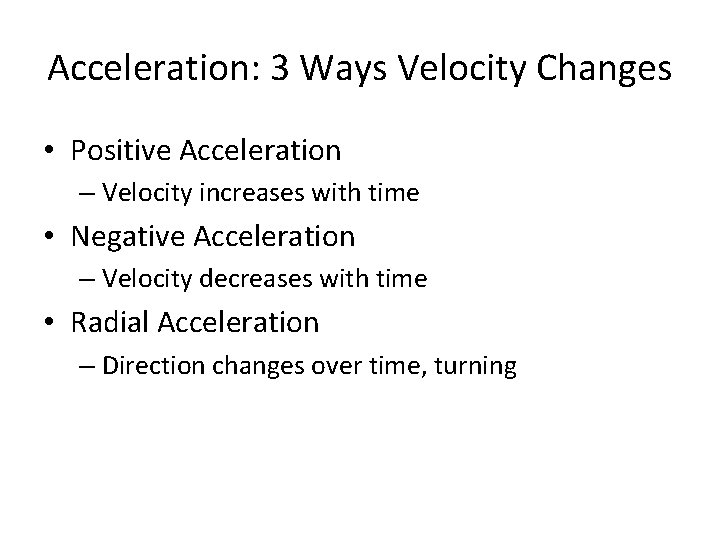Acceleration: 3 Ways Velocity Changes • Positive Acceleration – Velocity increases with time • Negative Acceleration – Velocity decreases with time • Radial Acceleration – Direction changes over time, turningBee's Velocity 30 Acceleration: 3 Ways Velocity Changes 20 • Positive acceleration Velocity (km/hr) 10 – Velocity increases with time • Negative acceleration 0 0 0. 05 0. 15 0. 25 0. 35 0. 45 0. 55 – Velocity decreases with time 0. 65 • Radial acceleration -10 – Direction of the velocity changes -20 -30 Time (hr) 0. 75 0. 85 0. 95 1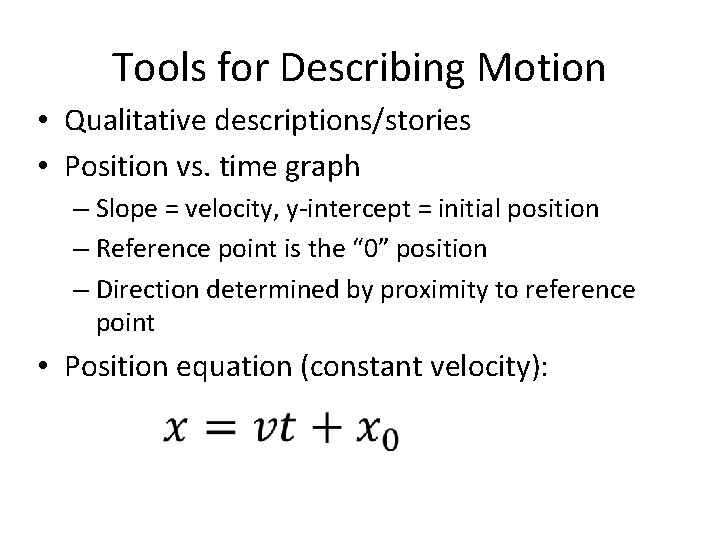Tools for Describing Motion • Qualitative descriptions/stories • Position vs. time graph – Slope = velocity, y-intercept = initial position – Reference point is the “ 0” position – Direction determined by proximity to reference point • Position equation (constant velocity):Tools for Describing Motion • Velocity vs. time graph – Slope = acceleration, y-intercept = initial velocity – Speed determined by proximity to “ 0” bar – Direction determined by quadrant of the graph – Area gives displacement • Velocity equation (constant acceleration): • Displacement equation (constant acceleration)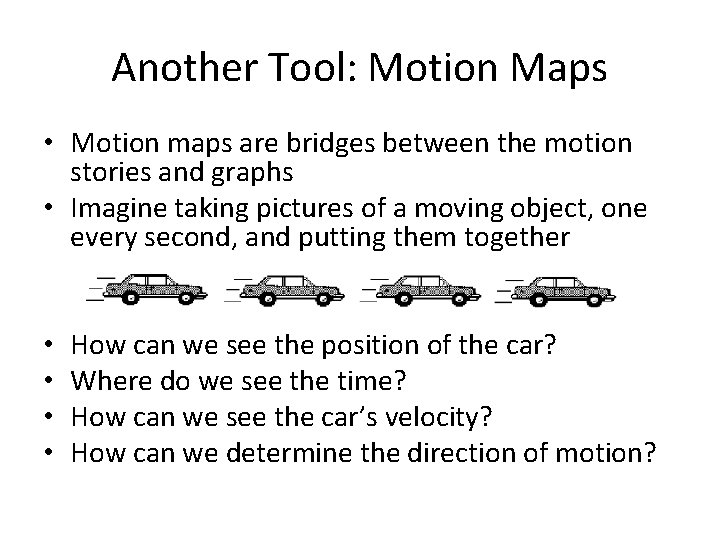Another Tool: Motion Maps • Motion maps are bridges between the motion stories and graphs • Imagine taking pictures of a moving object, one every second, and putting them together • • How can we see the position of the car? Where do we see the time? How can we see the car’s velocity? How can we determine the direction of motion?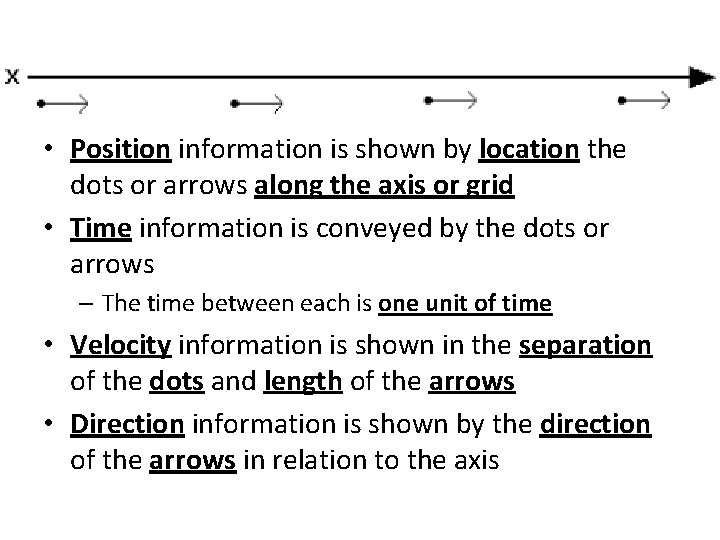• Position information is shown by location the dots or arrows along the axis or grid • Time information is conveyed by the dots or arrows – The time between each is one unit of time • Velocity information is shown in the separation of the dots and length of the arrows • Direction information is shown by the direction of the arrows in relation to the axis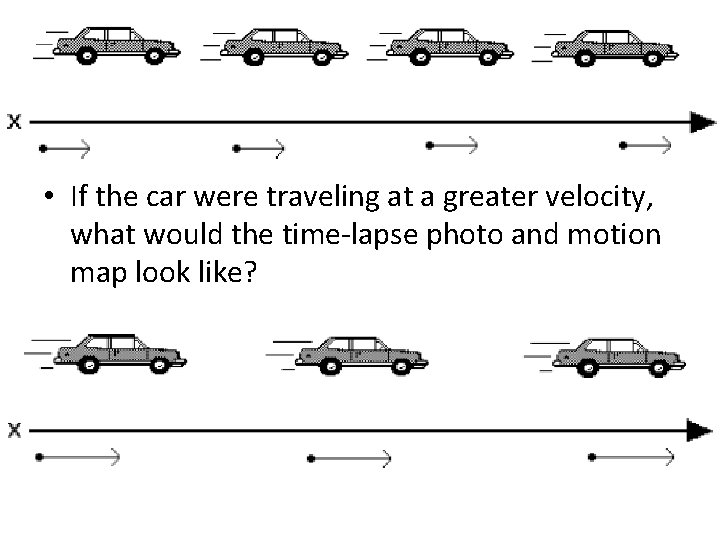• If the car were traveling at a greater velocity, what would the time-lapse photo and motion map look like?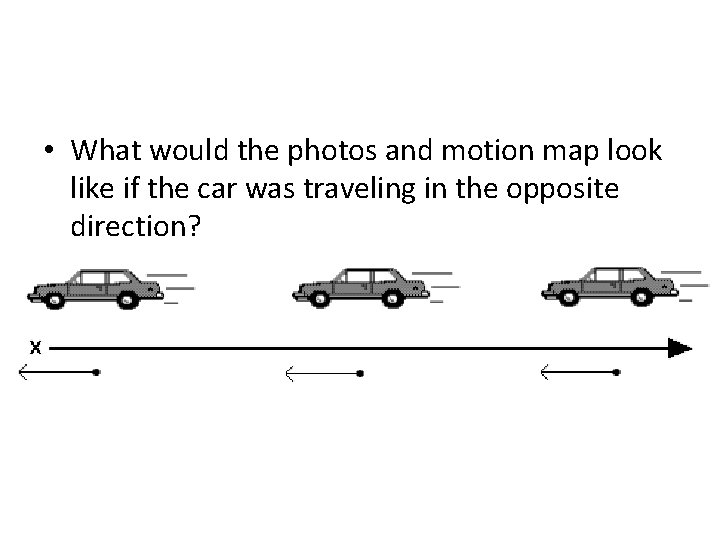• What would the photos and motion map look like if the car was traveling in the opposite direction?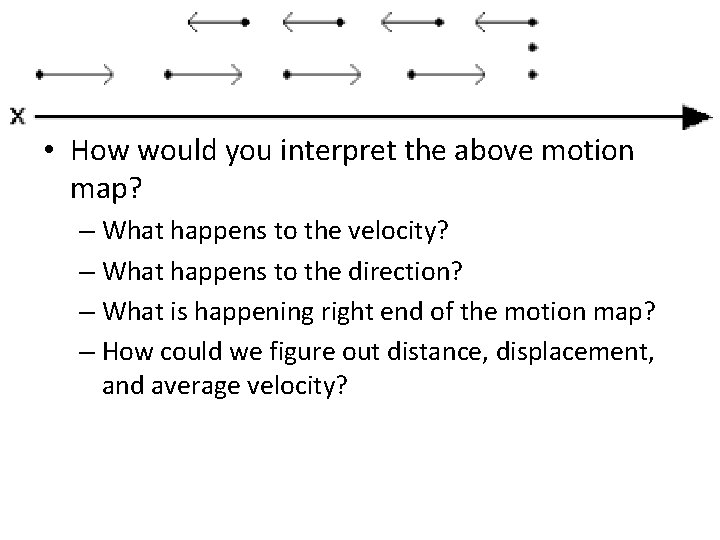• How would you interpret the above motion map? – What happens to the velocity? – What happens to the direction? – What is happening right end of the motion map? – How could we figure out distance, displacement, and average velocity?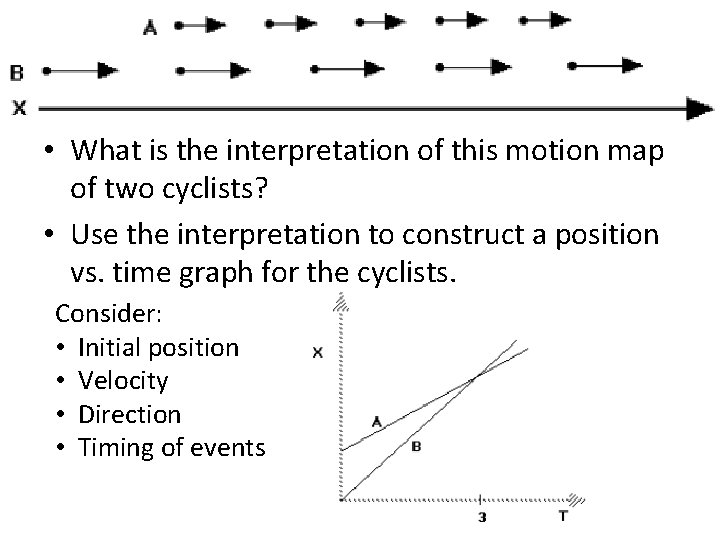• What is the interpretation of this motion map of two cyclists? • Use the interpretation to construct a position vs. time graph for the cyclists. Consider: • Initial position • Velocity • Direction • Timing of events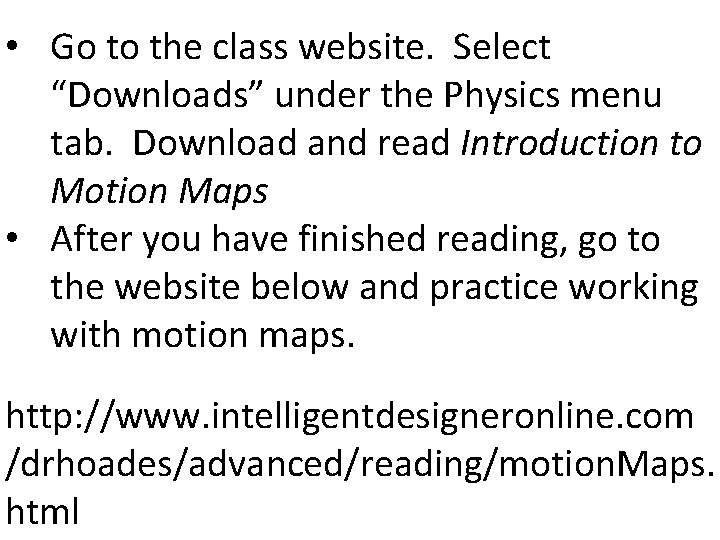• Go to the class website. Select “Downloads” under the Physics menu tab. Download and read Introduction to Motion Maps • After you have finished reading, go to the website below and practice working with motion maps. http: //www. intelligentdesigneronline. com /drhoades/advanced/reading/motion. Maps. html